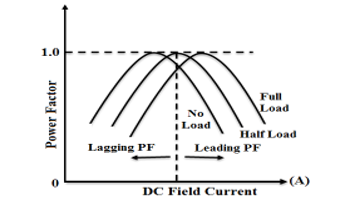Engineering Jobs   »   RVUNL ELECTRICAL 2021. SARKARI EXAM,

# RVUNL’21 EE: Daily Practices Quiz 31-Aug-2021

RVUNL Recruitment 2021: The RVUNL exam date is released for a total of 1075 vacancies.
There are two parts for the RVUNL test, Part A and Part B.
Part A will have 60% weightage while Part B has 40% weightage out of the total marks in the online written exam.

RVUNL’21 EE: Daily Practices Quiz 31-Aug-2021
Each question carries 2 marks.
Negative marking: 0.5 mark
Total Questions: 06

Time: 8 Min.

Q1. A 0 to 200 V voltmeter has a guaranteed accuracy of 0.5 percent of full-scale deflection. The voltage measured by the instrument is 50 V. Find the limiting error.
(a) 0.25 percent
(b) 1 percent
(c) 0.5 percent
(d) 2 percent
(e) 4 percent

Q2. Which of the following instruments has non-linear scale?
1. Moving coil instrument
2. Moving iron instrument
3. Electrostatic instrument
4. Rectifier instrument
Select the answer as per following codes:
(a) 2 only
(b) 2 and 3 only
(c) 2, 3 and 1
(d) 1, 2, 3 and 4
(e) None of the above

Q3. With reference to fault analysis, L-L fault is ____________ fault and the L-L-L-G fault is_________ fault.
(a) Unsymmetrical, Unsymmetrical
(b) Unsymmetrical, Symmetrical
(c) Symmetrical, Unsymmetrical
(d) Symmetrical, Symmetrical
(e) None of the above

Q4. A 3-phase 4 pole induction motor is supplied from a 50 Hz source. Find the rotor frequency when the rotor runs at 600 rpm.
(a) 20 Hz
(b) 25 Hz
(c) 50 Hz
(d) 30 Hz
(e) 40 Hz

Q5. For C coils and P poles, the distance between the coils connected by an equalizer ring is:
(a) C/2
(b) C/P
(c) C/2P
(d) 2C/P
(e) 4C/P

Q6. A synchronous motor is operating at constant load, while its excitation is adjusted to get unity power factor. If the excitation is now increased, the power factor will be?
(a) Remains at unity
(b) Becomes zero
(d) Lagging
(e) None of the above

SOLUTIONS

S1. Ans.(d)
Sol. % error=(0.5×200)/50=2 %

S2. Ans.(b)
Sol. Moving coil and Rectifier Instruments have linear scale. Moving iron and electrostatic have non-linear scale.

S3. Ans.(b)
Sol. Unsymmetrical faults:
L-L fault
L-G fault
L-L-G fault
Symmetrical faults:
L-L-L fault
L-L-L-G fault

S4. Ans.(d)
Sol. ACQ: N_r=600 rpm,N_S=(120×50)/4=1500 rpm
∴slip(s)=(1500-600)/1500=3/5=0.6
∴f_r=sf=0.6×50=30 Hz.

S5. Ans.(d)
Sol. The distance b/w equalizer ring(D)=2C/P

S6. Ans.(c)
Sol. If excitation increases for given condition, then Power factor becomes leading.Sharing is caring!

Thank You, Your details have been submitted we will get back to you.